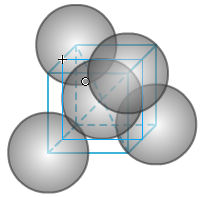#学习小专题

## 第二十二讲：晶体结构与性质（二）晶胞粒子数、空间利用率和密度ρ=M(g/mol)×{[b/(a×10－12m)3]/6.02×1023(/mol)}

=(Mb×1013)/(6.02×a3)g/m3

1、分子晶体的结构(1)晶胞中的分子数目计算：8×1/8＋6×1/2=4

(2)晶体密度计算：晶胞边长为572.265pm(计算数据)。

ρ=(Mb×1013)/(6.02×a3)g/m3

=(44×4×1013)/(6.02×572.2653) g/m3

=1560000g/m3=1.56g/ cm3

2、原子晶体的结构(1)晶胞中的原子数目计算：8×1/8＋6×1/2＋4=8

(2)空间利用率计算：根据两个C之间紧密相邻，设C的原子半径为1，则C－C为2。

(3)密度计算：以金刚石结构为例，C的共价半径为77pm。

ρ=12(g/mol)×{[8/(512/3√3)] ×(77×10－12m)3}/[6.02×1023(/mol)]

=3.545×106g/ m3=3.545 g/ cm3

4、金属晶体的结构

(1)简单立方①晶胞中原子数目计算：8×1/8=1。

②空间利用率计算：设球的半径为1，则正方体的边长为2，正方体的体积为8。

③密度计算：若为Po(钋)，原子半径为153pm，晶胞边长为336pm(资料数据)。则密度为

ρ=(Mb×1013)/(6.02×a3)g/m3

=(209×1×1013)/(6.02×3363)g/m3

=9.15 ×106g/m3=9.15g/cm3

(2)体心立方①晶胞中的原子数目计算：8×1/8＋1=2。

②空间利用率计算：在体心立方中，设为球半径为1，则正方体的体对角线为4，正方体的边长为4/√3。

③密度计算：若为Na，原子半径为186pm。

ρ=(Mb×1013)/(6.02×a3)g/m3

=(23×2×1013)/(6.02×429.563)g/m3

=9.64 ×105g/m3=0.964g/cm3

(3)面心立方①晶胞中的原子数目计算：8×1/8＋6×1/2=4。

②空间利用率计算：设球半径为1，则正方体的面对角线为4，正方体的边长为2√2。

③密度计算：若为Ag，原子半径为144pm。

ρ=(Mb×1013)/(6.02×a3)g/m3

=(108×4×1013)/(6.02×407.293)g/m3

=10.62 ×106g/m3=10.62g/cm3

(4)六方堆积①正六棱柱中原子数目计算：12×1/6＋2×1/2＋3=6

(晶胞为正六棱柱的1/3，则原子数目为2)

②空间利用率计算：设球的半径为1，则面上正六边形的边长为2，面积为6×√3=6√3。

(晶胞体积为正六棱柱的1/3，为8√2)

③密度计算：六方最密堆积不是正方体晶胞，不能用正方体的公式计算。

ρ={65.38g/mol×2/[8√2× (139×10－12m)3]}/(6.02×1023/mol)

=7.15×106g/ m3=7.15g/cm3

4、离子晶体的密度计算：

①NaCl：Na的离子半径为95pm，Cl的离子半径为181pmρ=(Mb×1013)/(6.02×a3)g/m3

=[(23×4＋35.45×4)×1013]/(6.02×5523)g/m3

=2.312 ×106g/m3=2.312g/cm3

②CsCl：Cs的离子半径为169pm，Cl的离子半径为181pm

ρ=(Mb×1013)/(6.02×a3)g/m3

=[(132.9＋35.45)×1013]/(6.02×4043)g/m3

=4.24 ×106g/m3=4.24g/cm3

③CaF2：Ca2＋的离子半径为99pm，F的离子半径为136pmρ=(Mb×1013)/(6.02×a3)g/m3

=[(40×4＋19×8)×1013]/(6.02×542.73)g/m3

=3.24 ×106g/m3=3.24g/cm3

【练习】

1、下图为CO2晶胞，一个晶胞中包含个CO2分子。2、金刚石的晶胞如下图所示，一个晶胞中包含个碳原子。3、计算下面的正六棱柱中有多少个球。。4、计算简单立方堆积的空间利用率。写出计算式：。5、若某金属的相对原子质量为X，原子半径为y pm，晶体中原子的堆积方式为简单立方，求此金属的密度。写出计算过程。

【练习答案】

1、4。

2、8。

3、6。

4、设球的半径为1，则正方体的边长为2，正方体的体积为8。

5、ρ=(Mb×1013)/(6.02×a3) g/m3

=(X×1×1013)/[6.02×(2y)3]g/m3

=4×1015X/301y3(g/m3)

(责任编辑：)

------分隔线----------------------------

------分隔线----------------------------

 难点十四：晶体的空间利用率和密 复习讲义：《凝练微粒堆积，洞悉 复习讲义：《凝练微粒堆积，洞悉 微课视频：晶体的空间利用率 微课视频：晶体密度的计算―以立 晶体密度计算专练 例析晶胞分割及晶体密度等参数的 微课视频：两种方法解CSCl晶体的 金属晶体空间利用率的计算与比较 晶体密度计算总结 浅谈金属晶体及堆积模型与空间利 氮化铝晶体密度计算方法 金刚石晶体密度计算方法 石墨晶体密度计算方法 晶体密度计算方法 NaCl型和CsCl型晶体其密度（D） 六方最密堆积晶体空间利用率的计• 全部评论（0
还没有评论，快来抢沙发吧！